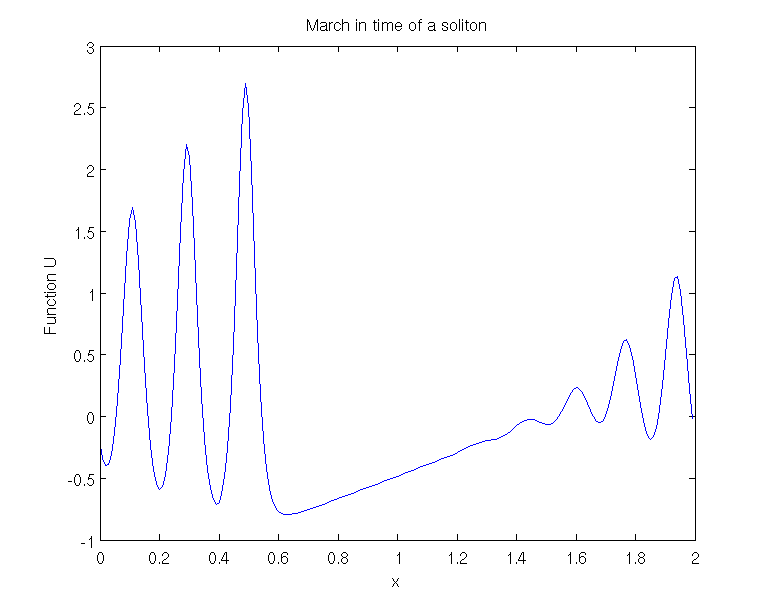# Solving of the equation of Korteweg - Vries (KDV)

Here we build a code that generates a solution to the equation of Korteweg and Vries. This code is based upon burgers_global.m This equation is : \displaystyle {\phi}_{t}+{\phi}^{3}_{x}+\phi 6{\phi}_{x}=0 The goal is to reproduce the figure in the page of this equation in wikipedia.

clear all; clf;

% parameters
Nx=200; % number of gridpoints
Lx=2; % domain length in x
delta=0.022;


On the beginning, we define all the matrix that will be used on the code. To do that we use the method of fourier written in fourdif.m.

% Construction of the three derivates on x
scale=Lx/(2*pi);
[x, d.x] = fourdif(Nx, 1);
[x, d.xx] = fourdif(Nx, 2);
[x, d.xxx] = fourdif(Nx, 3);

x=x*scale;
d.wx=ones(1,Nx)*(x(2)-x(1));
d.x=d.x/scale; d.xx=d.xx/scale^2;
d.xxx=d.xxx/scale^3;

x=x-x(1);

% system matrices
I=eye(Nx);
E=I;
A=-delta^2*d.xxx;


To reproduce exactly the figure on the wikipedia page, we do have to change a little the KDV equation. Thus we use the following equation : \displaystyle u_t + uu_x + {\delta}^{2}u_{xxx}=0 Just like in vibrating_string.m we use the Crank-Nicolson scheme for the linear terms, this gives \displaystyle M_m u(t+dt)=M_p u(t)-uu_x dt where the integral of the nonlinear term from t to t+dt was approximated by uu_xdt like in the forward Euler scheme, and with the matrices \displaystyle M_m=(E-Adt/2), M_p=(E+Adt/2)

% march in time matrix
dt=0.001;n=700;
t=0:dt:n*dt; Nt=length(t);

Mm=(E-A*dt/2);
M=Mm\(E+A*dt/2);


The initial condition used here is the following one : \displaystyle u(x,0)=cos(\pi x)

% initial condition
q=cos(pi*x);

% marching loop
for ind=1:(Nt-1)
nl=-q.*(d.x*q);

Here we should not forget that we have to impose the boundary conditions. Here the nonlinear terms act like a nonhomogeneous forcing. We should remember not to put a forcing on the boundary conditions. This would be good for nonhomogeneous boundary conditions, but this is not what we want to do here. So we put some zeros in the lines of the constraints (stored in loc). On the following code we have \displaystyle \text{The linear term is : } M*q \quad \text{and} \quad \text{the non linear term is : } \left(\frac{M_m}{nl}*dt\right)

    q=M*q+(Mm\nl*dt); % one step forward


# Validation of the code.

Here we do the plotting of the solution we found previously

plot(x,q);
title('March in time of a soliton');
axis([0,Lx -1 3]);
xlabel('x');
ylabel('Function U');
drawnow

end
set(gcf,'paperpositionmode','auto');
print('-dpng','-r120','korteweg_vries.png'); % save the figureThe plotting of the numerical solution